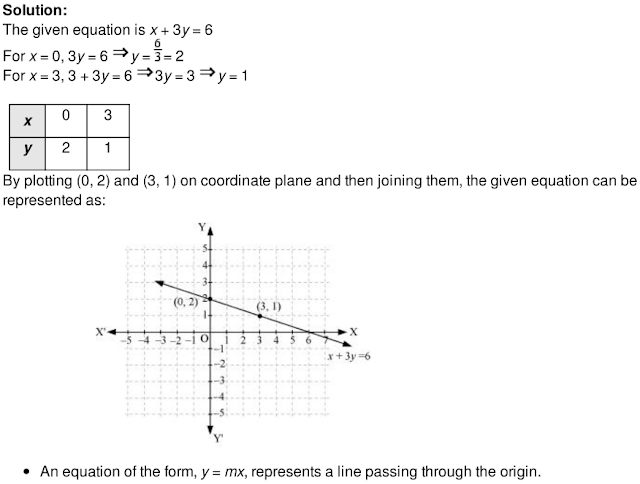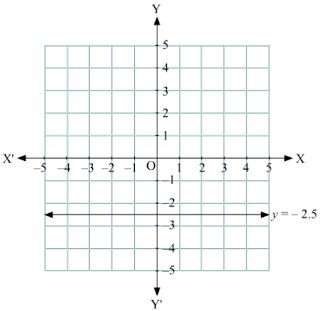## Revision Notes of Ch 4 Linear Equations in Two Variables Class 9th Math

Topics in the Chapter

• Linear equation in two variables
• Solution of a linear equation in two variables
• Representation of a linear equation in two variables graphically.
• Graphical solution of linear equation in two variables
• Represent the equation 2y + 5 = 0, on Cartesian plane.

Linear equation in two variables

An equation of the form, ax + by + c = 0, where a, b and c are constants, such that a and
b are both not zero and x and y are variables is called a linear equation in two variables.
For example, 2x + 3y + 10 = 0, 3x + 7y = 0
Real life situations can be expressed mathematically as linear equations in two variables.
Example:The age of Ram is 3 more than twice the age of Mohan. Write a linear equation in two
variables to represent this statement.
Solution:
Let the age of Mohan be x years and the age of Ram be y years.
Thus, the given condition can be expressed as y = 2x + 3

Solution of a linear equation in two variables

• The values of the variables in a linear equation, which satisfy the equation are the solutions of
that linear equation.

• A linear equation in two variables has infinitely many solutions.

• Solution of linear equation in two variables can be found by substitution method.

Example:
Find two different solutions of the equation 4x + 5y = 20.

Solution:

Given equation is 4x + 5y = 20.
If we take x = 0, we obtain:
4 × 0 + 5y = 20
⇒ 5y = 20
⇒ y = 4
So, (0, 4) is a solution of the given equation.
If we take y = 0, we obtain:
4x + 5 × 0 = 20
⇒ 4x = 20
⇒ x = 5
So, (5, 0) is a solution of the given equation.

Representation of a linear equation in two variables graphically.

The geometrical representation of the linear equation, ax + by + c = 0, is a straight line.
In order to represent a linear equation in two variables graphically, its two or three different
points are calculated and then the corresponding points are plotted and joined on the
coordinate plane.
Example:
Represent x + 3y = 6 on a graph paper.Graphical solution of linear equation in two variables

• Every point on the graph of a linear equation in two variables is a solution of the linear equation
and moreover, every solution of the linear equation is a point on the graph of the linear equation.

Example:A bag contains some Re 1 coins and some Rs 2 coins. The total worth of coins is Rs 45. Find the
number of Re 1 coins, if there are 10 coins of Rs 2.
Solution:
Let there be x coins of Re 1 and y coins of Rs 2.
Thus, 1x + 2y = 45
⇒ x + 2y = 45
This is the required linear equation of the given information. The three solutions of this equation
have been given in the tabular form as follows:From the above graph, it can be seen that the value of x corresponding to y = 10 is 25.
Therefore, there are 25 coins of Re 1, if there are 10 coins of Rs 2.
The graph of x = a is a straight line parallel to the y-axis, situated at a distance of a units
from y-axis.
The graph of y = b is a straight line parallel to the x-axis, situated at a distance of b units
from x-axis.

Example:
Represent the equation 2y + 5 = 0, on Cartesian plane.

Solution